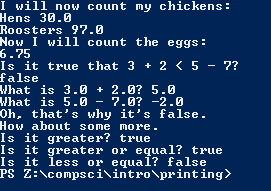# Assignment #11 NumbersAndMath

## Code

```        /// Name: Lacey Reese
/// Period: 5
/// Program Name: NumbersAndMath
/// File Name: NumbersAndMath.java
/// Date Finished 9/16/2015

public class NumbersAndMath
{
public static void main( String[] args )
{
System.out.println( "I will now count my chickens:" );

// 25 plus 30 divided by 6 is 30, so there are 30 hens, this line follows PEMDAS

System.out.println( "Hens " + ( 25.0 + 30.0 / 6.0 ) );

// you do 100 minus 25 and take the modulus of 4 and 3 and get 97, this follows PEMDAS

System.out.println( "Roosters " + ( 100.0 - 25.0 * 3.0 % 4.0 ) );

System.out.println( "Now I will count the eggs:" );

// you do 3 plus 2 plus 1 minus 5 plus 4 which is 5 and you add the modulus 2 and 1 and divide it by 10, follow PEMDAS

//3 plus 2 plus 1 minus 5 plus 4 which equals 5, then take the modulus of 4 and 2 and divide it by 6 plus 4 which is 10. follows PEMDAS

System.out.println( 3.0 + 2.0 + 1.0 - 5.0 + 4.0 % 2.0 - 1.0 / 4.0 + 6.0 );

// Is it true that 3 plus 2 is less than 5 minus 7?

System.out.println( "Is it true that 3 + 2 < 5 - 7?" );

// 3 plus 2 equals 5 and 5 minus 7 equals negative 2

System.out.println( 3.0 + 2.0 < 5.0 - 7.0 );

// What is 3 plus 2? thats 5, what is 5 minus 7? negative 2

System.out.println( "What is 3.0 + 2.0? " + ( 3.0 + 2.0 ) );

System.out.println( "What is 5.0 - 7.0? " + ( 5.0 - 7.0 ) );

// 5 in NOT less than negative 2, so that's why it is false

System.out.println( "Oh, that's why it's false." );

// One more problem

System.out.println( "How about some more." );

// Is 5 greater than negative 2? yes

System.out.println( "Is it greater? " + ( 5.0 > -2.0 ) );

// Is 5 greater or equal to negative 2? yes

System.out.println( "Is it greater or equal? " + ( 5.0 >= -2.0 ) );

// Is 5 less or equal to negative 2. no

System.out.println( "Is it less or equal? " + ( 5.0 <= -2.0 ) );
}
}

Picture of the output
// I have chickens!!!!!!!!!!```